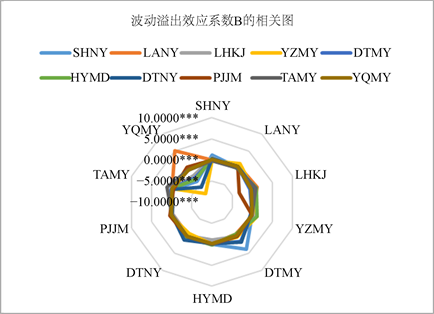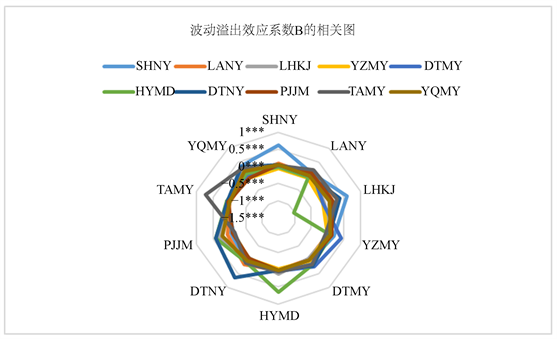# 上市公司“五性”间的波动溢出效应研究——基于面板及BEKK-GARCH模型的分析Research on the Fluctuation Spillover Effect of “Five Sex” in Listed Companies—Based on Panel and BEKK-GARCH Model Analysis

• 全文下载: PDF(1054KB)    PP.573-585   DOI: 10.12677/FIN.2019.96064
• 下载量: 180  浏览量: 340   国家自然科学基金支持

The supply-side structural reform proposed by China has transformed the form of economic de-velopment and changed the mode of production management. Therefore, it is of great significance to grasp the key characteristics of the listed company’s earnings and the dynamic changes of the volatility spillover effect in the context of supply-side reform. Based on the financial indicators of 18 major listed companies in China’s coal industry in 2004-2018, by constructing a panel regression model, the key factors affecting the growth of growth, liquidity, safety and productivity are identified, and the BEKK-GARCH model is built to exploit the profitability. The top 10 companies have dynamic changes in volatility spillovers in key characteristics. The results show that: (1) the key factor affecting profitability is productivity; (2) the productive volatility spillover effect in companies shows agglomeration, and these changes are related to reform policies; (3) large com-prehensive coal companies, such as Shenhua Energy can better grasp the key points of gains in the reform process, and its production and operation methods have guiding significance for other companies. Based on the research conclusions, this paper believes that enterprises should grasp the future development strategy of enterprises from the perspective of productivity.

1. 引言

2015年，为了应对中低端产品过剩、传统产业产能过剩、结构性的有效供给不足等问题，国家提出了供给侧结构性改革发展战略。改革要求各行业不仅要树立和贯彻落实创新、协调、绿色、开放、共享的发展理念，而且要在适度扩大总需求的同时，实行“三去一降一补”，即去产能、去库存、去杠杆、降成本、补短板，扩大有效供给，减少无效供给，提高生产效率。在此战略背景下，各行业都面临着改革的压力，煤炭行业更是首当其冲。众所周知，煤炭企业多表现为污染严重、高产能、高成本。我国煤炭行业在2016年产能过剩20亿吨左右，煤价下滑幅度较大，全行业亏损面达到了80%以上。供给侧结构性改革必然对煤炭行业的发展造成了巨大的冲击与影响。在供给侧结构性改革提出之后，煤炭行业中的一些龙头企业主动带头调整企业战略，重新布局生产与销售，重视环境保护，以满足改革的要求。为了促进改革在煤炭行业中的高速度高质量推进，了解供给侧结构性改革前后煤炭行业发生的变化，识别影响煤炭公司收益的关键因素，分析各企业之间波动溢出效应的动态变化关系就显得尤为重要。

2. 文献综述

3. 模型构建

3.1. 变量选择与数据说明

(一) 变量指标

$固定资产投入比率=\frac{固定资产}{总资产}×100%$

“五性”内部之间存在着一定的关系。企业的生产效率越高、安全环境越好、成长速度越快、资产变现能力越强对企业的发展越有利。企业发展情况的好坏可以直观的用企业的收益来显示出来。因此，可以将生产性、安全性、成长性、流动性作为自变量，探索其对收益性的影响。

(二) 样本数据说明

3.2. 面板数据模型

$\begin{array}{l}{\text{SYX}}_{it}={\alpha }_{i}+{\beta }_{1}{\text{CZX}}_{it}+{\beta }_{2}{\text{LDX}}_{it}+{\beta }_{3}{\text{AQX}}_{it}+{\beta }_{4}{\text{SCX}}_{it}+{\epsilon }_{it}\\ \text{\hspace{0.17em}}\text{\hspace{0.17em}}i=1,2,3,\cdots ,18;\text{\hspace{0.17em}}t=2004,2005,\cdots ,2018\end{array}$

3.3. BEKK-GARCH (1, 1)模型

4. 实证结果分析

4.1. 关键特性识别

(一) 描述性统计及相关检验Table 1. ADF unit root test for each indicatorTable 2. Descriptive statistics of five indicator sequences of 18 major coal listed companies

(二) 面板模型实证结果

$\begin{array}{l}{\text{SYX}}_{it}=-0.7+{\alpha }_{i}^{\ast }+0.0594{\text{CZX}}_{it}+0.006{\text{LDX}}_{it}+0.0908{\text{AQX}}_{it}+0.1832{\text{SCX}}_{it}\\ \text{\hspace{0.17em}}\text{\hspace{0.17em}}\text{\hspace{0.17em}}\text{\hspace{0.17em}}\text{\hspace{0.17em}}\text{\hspace{0.17em}}\text{\hspace{0.17em}}\text{\hspace{0.17em}}\text{\hspace{0.17em}}\text{\hspace{0.17em}}\text{\hspace{0.17em}}\left(\ast \ast \ast \right)\text{\hspace{0.17em}}\text{\hspace{0.17em}}\text{\hspace{0.17em}}\text{\hspace{0.17em}}\text{\hspace{0.17em}}\text{\hspace{0.17em}}\text{\hspace{0.17em}}\text{\hspace{0.17em}}\text{\hspace{0.17em}}\text{\hspace{0.17em}}\text{\hspace{0.17em}}\text{\hspace{0.17em}}\left(\ast \ast \ast \right)\text{\hspace{0.17em}}\text{\hspace{0.17em}}\text{\hspace{0.17em}}\text{\hspace{0.17em}}\text{\hspace{0.17em}}\text{\hspace{0.17em}}\text{\hspace{0.17em}}\text{\hspace{0.17em}}\text{\hspace{0.17em}}\text{\hspace{0.17em}}\text{\hspace{0.17em}}\text{\hspace{0.17em}}\text{\hspace{0.17em}}\text{\hspace{0.17em}}\text{\hspace{0.17em}}\text{\hspace{0.17em}}\text{\hspace{0.17em}}\text{\hspace{0.17em}}\text{\hspace{0.17em}}\text{\hspace{0.17em}}\text{\hspace{0.17em}}\text{\hspace{0.17em}}\text{\hspace{0.17em}}\text{\hspace{0.17em}}\text{\hspace{0.17em}}\text{\hspace{0.17em}}\text{\hspace{0.17em}}\text{\hspace{0.17em}}\text{\hspace{0.17em}}\text{\hspace{0.17em}}\text{\hspace{0.17em}}\text{\hspace{0.17em}}\text{\hspace{0.17em}}\text{\hspace{0.17em}}\text{\hspace{0.17em}}\text{\hspace{0.17em}}\text{\hspace{0.17em}}\text{\hspace{0.17em}}\text{\hspace{0.17em}}\text{\hspace{0.17em}}\text{\hspace{0.17em}}\text{\hspace{0.17em}}\text{\hspace{0.17em}}\text{\hspace{0.17em}}\text{\hspace{0.17em}}\text{\hspace{0.17em}}\text{\hspace{0.17em}}\text{\hspace{0.17em}}\text{\hspace{0.17em}}\text{\hspace{0.17em}}\text{\hspace{0.17em}}\text{\hspace{0.17em}}\left(\ast \ast \ast \right)\\ i=1,2,3\cdots ,18;\text{\hspace{0.17em}}\text{\hspace{0.17em}}t=2004,2005,\cdots ,2018\end{array}$ (1)Table 3. Fixed effects of individual sections (partial data)

Fixed Effects (Cross)是指每个截面公司的收益性表现相对于平均收益性表现(即常数项C)的偏离，反映了每个截面公司之间收益表现的结构差异。通过计算发现每个截面公司的收益性表现存在着显著差异。其中，中国神华能源股份有限公司的收益性表现水平最高，山西潞安环保能源开发股份有限公司次之，收益性表现水平排名第三位的为山西兰花科技创业股份有限公司，收益性水平表现均为正值。中国神华是一家以煤炭为基础的大型综合性公司，是我国上市公司中最大的煤炭销售商，拥有最大规模的煤炭储量，其在2017年和中国国电集团公司合并重组成为国家煤炭集团，改革任务艰巨，实证结果也表明中国神华在样本范围煤炭公司中收益性表现最为突出。表4列出了收益性表现前10名的公司。Table 4. Top 10 major coal listed companies with income levels

4.2. 波动溢出效应及特征分析

4.2.1. 相关检验

(一) 平稳性检验

(二) 残差序列的ARCH检验

$\begin{array}{l}D\left({\text{SCX}}_{it}\right)={\alpha }_{i}+{\beta }_{i}D\left({\text{SCX}}_{i,t-1}\right)+{u}_{t}\\ i=1,2,3,\cdots ,18;\text{\hspace{0.17em}}\text{\hspace{0.17em}}t=2004,2005,\cdots ,2018\end{array}$Table 5. ADF unit root test for productive sequences (partial data)Table 6. ARCH-LM test of residuals (partial data)

4.2.2. 波动溢出效应

(一) 第一阶段波动溢出效应Table 7. Parameter estimation results of the first stage BEKK-GARCH (1, 1) modelFigure 1. Correlation diagram of inter-company productivity fluctuation effect coefficient B from 2004 to 2015

(二) 第二阶段波动溢出效应Table 8. Parameter estimation results of the second stage BEKK-GARCH (1, 1) modelFigure 2. Correlation diagram of inter-company productivity fluctuation effect coefficient B in 2004-2018

5. 结论

NOTES

*通讯作者。

  郭栋. 美国国债利率对中国债市宏观基本面冲击及两国利率联动时变效应研究——基于GVAR和TVP-VAR模型的实证分析[J]. 国际金融研究, 2019, 384(4): 55-65.  冯其云, 周靖祥. 高铁时代广深双城及其与腹地城市联动关系识别的证据[J]. 重庆大学学报(社会科学版), 2017, 23(2): 15-29.  杨文华, 卢露, 林树. P2P网贷利率与传统金融市场利率的联动效应研究——基于尾部风险溢出视角[J]. 金融经济学研究, 2018, 33(5): 61-70.  陈暮紫, 陈敏, 吴武清, 缪柏其. 中国A股市场行业板块间领滞关系的动态变化实证研究[J]. 系统工程理论与实践, 2009, 29(6): 18-31  蒋勇, 王国长, 吴武清. 股指期货的价格发现机制:基于结构变点分析框架[J]. 财经科学, 2014(6): 52-62.  刘凤根, 周驭舰. 股票市场波动性特征及溢出效应经验研究[J]. 云南财经大学学报, 2018, 34(11): 47-58.  谭小芬, 张峻晓, 郑辛如. 国际大宗商品市场与金融市场的双向溢出效应——基于BEKK-GARCH模型和溢出指数法的实证研究[J]. 中国软科学, 2018(8): 31-48.  林征, 林雅娜, 黄晓玲. 黄金、美元、石油市场间溢出效应研究——基于3元BEKK-GARCH模型的实证分析[J]. 经济研究导刊, 2015(11): 186-189.  何红霞, 胡日东. 大中华区股市波动溢出效应实证研究——基于多元非对称BEKK-GARCH模型[J]. 重庆科技学院学报(社会科学版), 2011(10): 139-143.  楚尔鸣, 鲁旭. 基于VECM-BEKK-二元GARCH模型的沪市A、B股市场信息传导关系研究[J]. 经济评论, 2009(2): 50-56.  孙少岩, 孙文轩. 加入SDR后人民币汇率波动规律研究——基于ARIMA-GARCH模型的实证分析[J]. 经济问题, 2018, 474(2): 42-47.  黄飞雪, 谷静, 李延喜, 苏敬勤. 金融危机前后的全球主要股指联动与动态稳定性比较[J]. 系统工程理论与实践, 2010, 30(10): 1729-1740.  谭海霞. 煤炭业安全经济贡献率测算及安全投入的社会分工[D]: [博士学位论文]. 重庆: 重庆大学, 2011.  Bollerslev, T., Engle, R.F. and Wooldridge, J.M. (1888) A Capital Asset Pricing Model with Time-Varying Covariances. Journal of Political Economy, 96, 116-131. https://doi.org/10.1086/261527  Engle, R.F. and Kroner, K.F. (1895) Multivariate Simul-taneous Generalized ARCH. Econometric Theory, 11, 122-150. https://doi.org/10.1017/S0266466600009063